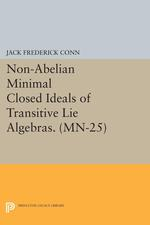Have library access? Log in through your library# Non-Abelian Minimal Closed Ideals of Transitive Lie Algebras. (MN-25):

JACK FREDERICK CONN
Copyright Date: 1981
Pages: 228
https://www.jstor.org/stable/j.ctt7ztv3j

## Table of Contents

1. Front Matter
(pp. [i]-[ii])
2. Table of Contents
(pp. [iii]-[iv])
3. Preface
(pp. [v]-[vi])
Jack F. Conn
4. Introduction
(pp. 1-14)

Transitive pseudogroups of local diffeomorphisms preserving geometric structures on manifolds have been studied by many authors; the origins of this subject are classical, and may be said to lie in the works of Sophus Lie and Élie Cartan. The structure of such a pseudogroup Γ acting on a manifold X is reflected in the structure of the Lie algebra of formal infinitesimal transformations of Γ, that is to say, those formal vector fields on X which are formal solutions to the linear partial differential equation which defines the infinitesimal transformations of Γ. The Lie algebras of formal vector fields obtained...

5. 1. Preliminaries
(pp. 15-64)

Throughout this section, we denote by K an arbitrary field of characteristic zero which is endowed with the discrete topology.

Let V be a Hausdorff topological vector space over K. Then V is said to be linearly compact if:

(i) V is complete; and

(ii) There exists a fundamental system {Vα} of neighborhoods of 0 in V such that each Vαis a vector subspace of finite codimension in V.

An example of such a space is provided by the local algebra$\text{F}=\text{K}[[{{\text x}_{1}},\ \ldots \ ,{{\text{x}}_{\text n}}]]$of formal power series over K in n indeterminates x1, … , xn. Denote In by F0...

6. 2. Derivations of Transitive and Simple Lie Algebras
(pp. 65-94)

Throughout this section, we denote by K a field of characteristic zero.

Let L be a Lie algebra over K, and let A be a subalgebra of L. By a derivation of A into L, we shall mean a K-linear mapping$\overline{\text{D}}:\text{A}\to \text{L}\ ,$such that, for all ξ,$\eta \ \epsilon \ \text{A}$$\overline{\text{D}}([\xi,\eta ])=[\overline{\text{D}}(\xi),\eta ]+[\xi,\overline{\text{D}}(\eta )]\ ;$from the Jacobi identity in L, we see that if$\zeta\ \epsilon \ \text{L}$, then ad(ζ) is a derivation of A into L. When A = L, such a linear mapping$\overline{\text{D}}$is simply a derivation of L. Recall that, in the last section, we established the notation Der(R) for the Lie algebra...

7. 3. Simple Algebras with Parameters
(pp. 95-109)

Let L be a linearly compact Lie algebra over a field K of characteristic zero. Suppose that V is a finite-dimensional vector space over K. We denote by F the linearly compact local algebra$\text{F}=\text{F}\{{{\text{V}}^{*}}\}$of formal power series on V, and we set${{\text{F}}^{l}}={{\text{F}}^{l}}\{{{\text V}^{*}}\}\ ,\quad \quad \quad \text{for}\ l\ge -1\ .$Recall that the completed tensor product$\text{L}{{\hat{\otimes }}_{\text K}}\ \text{F}$, defined in Section one, is a linearly compact vector space over K. There is a natural structure of Lie algebra on the dense subspace$\text{L}{{\otimes }_{\text{K}}}\ \text{F}$of$\text{L}{{\hat{\otimes }}_{\text K}}\ \text{F}$, defined by setting$\caption {(3.1)} [\xi \otimes \text{f},\eta \otimes \text{g}]=[\xi,\eta ]\otimes (\text{fg})\ ,$for all ξ,$\eta \ \epsilon \ \text{L}$and f,$\text{g}\ \epsilon \ \text{F}$. One verifies easily that there is a...

8. 4. Closed Ideals of Transitive Lie Algebras
(pp. 110-151)

To increase the intelligibility of this section, we have reproduced, in Lemma 4.1 through Corollary 4.3, certain technical results from the paper () of Guillemin.

Let L be a linearly compact Lie algebra over a field K of characteristic zero, and let I be a non-zero closed ideal of L. According to (ii) of Lemma 1.6, there exists a closed ideal J of I which is properly contained in I and strictly maximal. Since J is closed in L, the normalizer NL(J) of J in L is a closed subalgebra of L. The first series of lemmas of this section...

9. 5. Minimal Closed Ideals of Complex Type
(pp. 152-216)

Throughout this section, if V is a linearly compact topological vector space over${\rm I\!R}$, we denote by${{\text{V}}_{\mathbb{C}}}=\mathbb{C}{{\otimes }_{\rm{I\!R}}}\text{V}$the complexification of V; then${{\text{V}}_{\mathbb{C}}}$, is a linearly compact complex topological vector space.

Let L be a linearly compact Lie algebra over${\rm I\!R}$, and let I be a non-abelian minimal closed ideal of L. Recall that, by Corollary 4.3, there exists a unique maximal closed ideal J of I, and the quotient

R = I/J

is a non-abelian simple transitive Lie algebra. According to Proposition 1.4, the commutator ring KRof R is a field which is a...

10. References
(pp. 217-220)
11. Back Matter
(pp. 221-222)